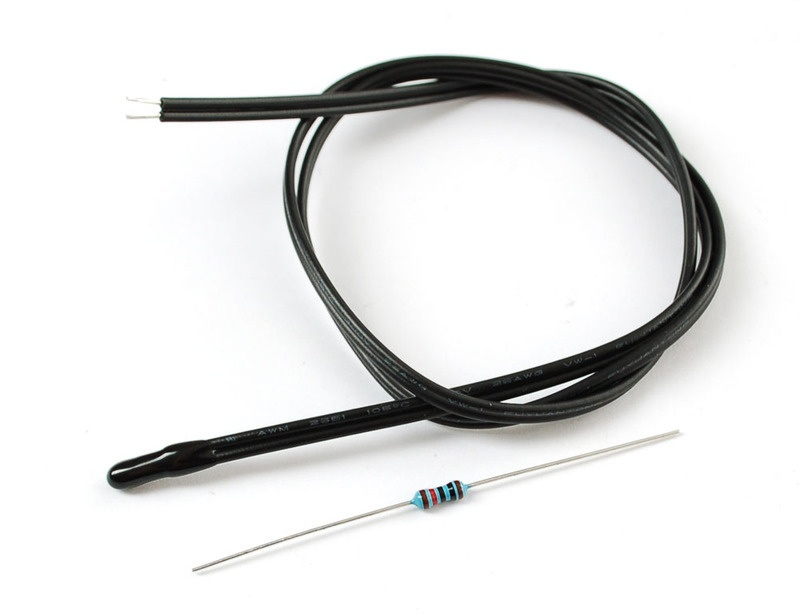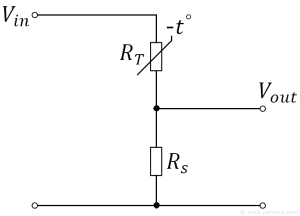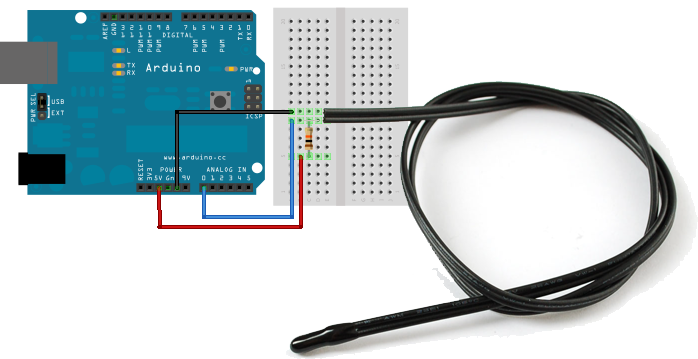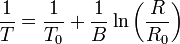##### Page tree
Go to start of banner

# Overview

A thermistor is a thermal resistor - a resistor that changes its resistance with temperature. Technically, all resistors are thermistors - their resistance changes slightly with temperature - but the change is usually very very small and difficult to measure. Thermistors are made so that the resistance changes drastically with temperature so that it can be 100 ohms or more of change per degree!There are two kinds of of thermistors, NTC (negative temperature coefficient) and PTC (positive temperature coefficient). In general, you will see NTC sensors used for temperature measurement. PTC's are often used as resettable fuses - an increase in temperature increases the resistance which means that as more current passes through them, they heat up and 'choke back' the current, quite handy for protecting circuits!

Thermistors have some benefits over other kinds of temperature sensors such as analog output chips (LM35/TMP36) or digital temperature sensor chips (DS18B20) orthermocouples.

• First off, they are much much cheaper than all the above! A bare 5% thermistor is only 10 cents in bulk.
• They are also much easier to waterproof since its just a resistor.
• They work at any voltage (digital sensors require 3 or 5V logic).
• Compared to a thermocouple, they don't require an amplifier to read the minute voltages - you can use any microcontroller to read a thermistor.
• They can also be incredibly accurate for the price. For example, the 10K 1% thermistor in the shop is good for measuring with ±0.25°C accuracy! (Assuming you have an accurate enough analog converter)
• They are difficult to break or damage - they are much simpler and more reliable

On the other hand, they require a little more work to interpret readings, and they dont work at very high temperatures like thermocouples. Without a digital-to-analog converter on board, you might be better off with a digital temperature sensor.

Their simplicity makes them incredibly popular for basic temperature feedback control. For example, lets say you wanted to have a fan that turns on when the temperature gets high. You could use a microcontroller, a digital sensor, and have that control the relay. Or you could use the thermistor to feed the base of a transistor, as the temperature rises, the resistance goes down, feeding more current into the transistor until it turns on. (This is a rough idea, you would need a few more components to make it work)

Even if you do use a microcontroller or complex system, for the price you can't beat 'em!

# Wiring

We can use a simple voltage divider circuit to measure the voltage across the thermistor.Vout = Rt / (Rt + Rs) * Vin

# Calculating Rt

Say the fixed resistor is 10K and the variable resistor is called R - the voltage output (Vo) is:

Vout = Rt / (Rt + 10K) * VinRemember that when you measure a voltage (Vi) into an Arduino ADC, you'll get a number.

ADC value = Vi * 1023 / Varef

So now we combine the two (Vout = Vi) and get:

ADC value = Rt / (Rt + 10K) * Vcc * 1023 / Varef

What is nice is that if you notice, if Vcc (logic voltage) is the same as the ARef, analog reference voltage, the values cancel out!

ADC value = Rt / (Rt + 10K) * 1023

Finally, what we really want to do is get that Rt (the unknown resistance). So we do a little math to move the Rt to one side:

Rt = 10K / (1023/ADC - 1)

```// thermistor-1.ino Simple test program for a thermistor for Adafruit Learning System

// the value of the 'other' resistor
#define SERIESRESISTOR 10000

// What pin to connect the sensor to
#define THERMISTORPIN A0

void setup(void) {
Serial.begin(9600);
}

void loop(void) {

// convert the value to resistance
Serial.print("Thermistor resistance ");

delay(1000);
}```

# Computing Temperature

We'll use the Steinhart-Hart equation , which lets us do a good approximation of converting values.

Its not as exact as the thermistor table (it is an approximation) but its pretty good around the temperatures that this thermistor is used.

Simplified EquationCode

```// Thermistor Example #3 from the Adafruit Learning System guide on Thermistors

// which analog pin to connect
#define THERMISTORPIN A0
// resistance at 25 degrees C
#define THERMISTORNOMINAL 10000
// temp. for nominal resistance (almost always 25 C)
#define TEMPERATURENOMINAL 25
// how many samples to take and average, more takes longer
// but is more 'smooth'
#define NUMSAMPLES 5
// The beta coefficient of the thermistor (usually 3000-4000)
#define BCOEFFICIENT 3950
// the value of the 'other' resistor
#define SERIESRESISTOR 10000

int samples[NUMSAMPLES];

void setup(void) {
Serial.begin(9600);
analogReference(EXTERNAL);
}

void loop(void) {
uint8_t i;
float average;

// take N samples in a row, with a slight delay
for (i=0; i< NUMSAMPLES; i++) {
delay(10);
}

// average all the samples out
average = 0;
for (i=0; i< NUMSAMPLES; i++) {
average += samples[i];
}
average /= NUMSAMPLES;

Serial.println(average);

// convert the value to resistance
average = 1023 / average - 1;
average = SERIESRESISTOR / average;
Serial.print("Thermistor resistance ");
Serial.println(average);

float steinhart;
steinhart = average / THERMISTORNOMINAL;     // (R/Ro)
steinhart = log(steinhart);                  // ln(R/Ro)
steinhart /= BCOEFFICIENT;                   // 1/B * ln(R/Ro)
steinhart += 1.0 / (TEMPERATURENOMINAL + 273.15); // + (1/To)
steinhart = 1.0 / steinhart;                 // Invert
steinhart -= 273.15;                         // convert to C

Serial.print("Temperature ");
Serial.print(steinhart);
Serial.println(" *C");

delay(1000);
}```

• No labels